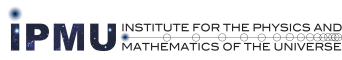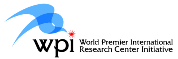### IPMU Seminar on Mathematical Physics

 Date: Monday, December 17 17:00 - 18:30 Place: Room 002, Mathematical Sciences Building Komaba Campus, The University of Tokyo Lecturer: Ken-Ichi Yoshikawa (The University of Tokyo) Title: Analytic torsion for Calabi-Yau threefolds Abstract: In 1994, Bershadky-Cecotti-Ooguri-Vafa conjectured that analytic torsion gives rise to a function on the moduli space of Calabi-Yau threefolds and that it coincides with the quantity \$F_{1}\$ in string theory. Since the holomorphic part of \$F_{1}\$ is conjecturally the generating function of the counting problem of elliptic curves in the mirror Calabi-Yau threefold, this implies the conjectural equivalence of analytic torsion and the counting problem of elliptic curves for Calabi-Yau threefolds through mirror symmetry. After Bershadsky-Cecotti-Ooguri-Vafa, we introduced an invariant of Calabi-Yau threefolds, which we obtained using analytic torsion and a Bott-Chern secondary class. In this talk, we will talk about the construction and some explicit formulae of this analytic torsion invariant. Some part of this talk is based on the joint work with H. Fang and Z. Lu.